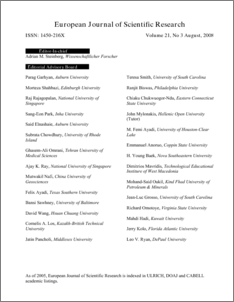# Fixed Coefficients Block Backward Differentiation Formulas for the Numerical Solution of Stiff Ordinary Differential Equations

## Citation

Ibrahim, Zarina Bibi and Suleiman, Mohamed and Othman, Khairil Iskandar (2008) Fixed Coefficients Block Backward Differentiation Formulas for the Numerical Solution of Stiff Ordinary Differential Equations. European Journal of Scientific Research, 21 (3). pp. 508-520. ISSN 1450-216X

## Abstract

This paper focuses on the derivation of implicit 2-point block method based on Backward Differentiation Formula (BDF) which will be called BBDF of variable step size for solving first order stiff initial value problems (IVPs) for Ordinary Differential Equations (ODEs). The method presented is similar to the form of standard BDF. This allows us to store the coefficients of the y values and thus avoiding calculating the differentiation coefficients at each step but robust enough to allow for step size variation.Plots of their regions of absolute stability for the method are also presented. The efficiency of the 2-point BBDF is compared with the conventional variable step variable order BDF(VSVOBDF) method. Numerical results indicate that the resulting 2-point BBDF method outperform the VSVOBDF method in both execution time and accuracy.Preview
PDF
114.pdfView Item